Next: Exercises Up: Background Previous: Exponentials and Logarithms

### Inverse functions

Consider the functions f, g defined for any real x by: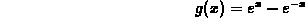You can plot the functions to get a hint to whether they are invertible or not. on the interval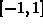.

```  > f:=x->E^x+E^(-x);
``````  > plot(f(x),x=-1..1);
```

```  > g:=x->E^x-E^(-x);
```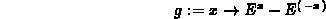```  > plot(g(x),x=-1..1);
```

We observe that f is not invertible since it does not satisfy the horizontal line test. Indeed f is not one-to-one since, for instance,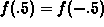. From the plot, it seems that the function g satisfies the horizontal line test. In order to determine its inverse, we solve for x: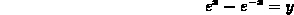```  > solve(E^x-E^(-x)=y,x);
```We observe that one of the solutions is not defined since the argument of the logarithm can only be positive. Thus:Let us check that we computed the right inverse. By definition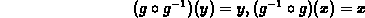Indeed if we denote the inverse function by ginv and compose the functions we get:

```  > ginv:=y->ln(1/2*y+1/2*sqrt(y^2+4));
```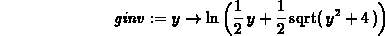```  > (g@ginv)(y);
```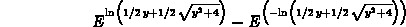```  > simplify((g@ginv)(y));
```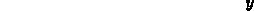```  > (ginv@g)(x);
```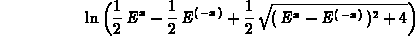```  > simplify((ginv@g)(x));
```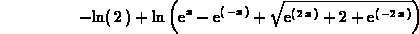You have do some manipulations in the last output to obtain x !

Sean O Anderson
Tue Nov 28 15:50:23 EST 1995